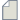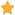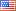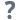###Author Topic: COSMO -organic solvents  (Read 35 times)

0 Members and 1 Guest are viewing this topic.

####KHipps

• New ATK user
•• Posts: 1
• Country:• Reputation: 0##### COSMO -organic solvents
« on: January 16, 2023, 12:52 »
I have been estimating solvation using the new COSMO functionality in ATK and I am having some issues with understanding what is actually being done and then finding that the answers do not compare well with either experiment or SMD calculations in Gaussian.  In particular:

1)  The basic COSMO calculator requires both dielectric constant and surface tension for the solvent.  The primary reference given in a recent ATK tutorial was Klamt, J Comput. Chem 21, 1648, 2018.  This paper discusses only the electrostatic correction and does not include the work done to form the surface (surface tension term).  This suggests that the actual calculation does more than just the electrostatic correction and might estimate the free energy of solvation.  Does anyone know what is actually being calculated and what the correct citation(s) are?

2)  If one calculates the COSMO "solvation energy" for n-hexane as a molecule in toluene or benzene, one gets (respectively) -3.2 and -2.0 kcal/mole.  The SMD method in Gaussian 16 gives solvation free energies of -4.08 and -4.24 kcal/mole, respectively.  The experimental value for hexane in benzene is -3.6.
For this limited test, the values produced by the cosmo "surface energy" are roughly correct for the free energy.
It gets weird if one adds a COSMO-RS calculation to the work flow.  For hexane in either toluene or benzene the reported "surface energy" is only about 0.4 kcal/mole!

3) when one uses a slab model COSMO calculation, the results are strange.  For the 0001 face of graphite in phenyloctane, one finds a "solvation energy" of -19.3 ergs/cm^2.  This is about 5 times less than experiment!  For a Au 111 surface in n-hexane, a value of -26.3 ergs/cm^2 is obtained.  While I haven't been able to find an experimental value for this, my reading suggests that it is also about one order too small.

Any help in making sense of all this would be greatly appreciated.